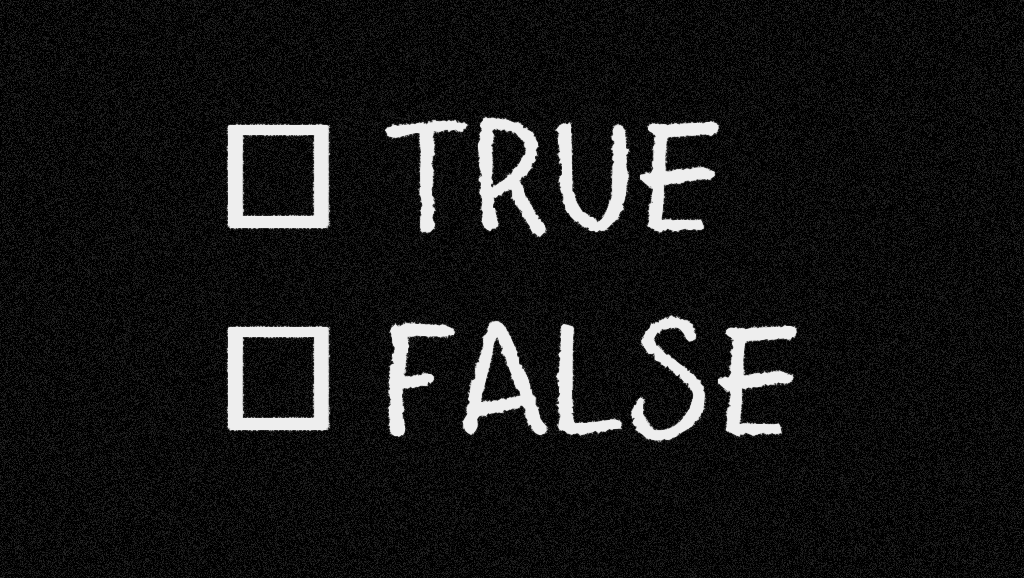# True & False riddle

747.5K Views

Consider a list of 2000 statements:

1) Exactly one statement on this list is false.
2) Exactly two statements on this list are false.
3) Exactly three statements on this list are false.
. . .
2000) Exactly 2000 statements on this list are false.
Which statements are true and which are false?

What happens if you replace “exactly” with “at least”?

What happens if you replace “exactly” with “at most”?

What happens in all three cases if you replace “false” with “true”?The first riddle is simple.
All statements are mutually exclusive.
If statement 20 would be true all other statements would be false.
Which means that there can only be one true statement and 1999 false statements.
So statement 1999 “Exactly 1999 statements on this list are false.” is true.

replace “exactly” with “at least”
If statement X is true then there are at least X false statements.
Which means that there are also at least X-1 statements false and that makes statement X-1 also true.
So then X statements are true and at least X statements are false.
Because the total number of statements is 2000, X will be 1000.
The statements 1 to 1000 are true and 1001 to 2000 are false.

replace “exactly” with “at most”
Statement 2000 is true because with only 2000 statements there can be at most 2000 false statements.
If statement X and all statements after that are true then there are at most X-1 false statements which means that statement X-1 is also true.
This means that all statements before X are also true.
So all statements are true. (no false statements meets the condition of “at most X are false”.)

replace “false” with “true”
The statements are still all mutually exclusive.
So only one statement is true and that is the first statement.

replace “false” with “true” and “exactly” with “at least”
If statement X is false then all statements after X must be false because there are at most X-1 true statements.
That also means that when statement X is true all statements before statement X must be true.
And here have a problem.
If we assume that statements 2 to 2000 are all false and only look at statement 1.
If statement 1 is true than there is “at least one true statement”
But if statements 1 is false there is not “at least one true statement”
Both are solutions of the riddle.
So there are 2001 good solutions.

replace “false” with “true” and “exactly” with “at most”
If statement X is true then there are at most X true statements.
Which means that there are also at most X+1 statements true and that makes statement X+1 also true.
So there are at most X true statements but also at least (2001 – X) true statements.
The statements 1 to 1000 are false and 1001 to 2000 are true.